## Indicators

•Cited by SciELO
•Access statistics

•Cited by Google
•Similars in SciELO
•Similars in Google

## Print version ISSN 0123-3033

### Ing. compet. vol.16 no.1 Cali Jan./June 2014

Arquitectura hardware para la implementación de la transformada discreta Wavelet 2D

Hardware Architecture for the Implementation of the Discrete Wavelet Transform in two Dimensions

Norma X. Ríos-Cotazo
Facultad de Ingeniería, Institución Universitaria Antonio José Camacho, Cali, Colombia
E-mail: ximena.rios.cot@gmail.com

Álvaro Bernal-Noreña
Grupo GADyM, Escuela de Ingeniería Eléctrica y Electrónica, Universidad del Valle, Cali, Colombia
E-mail: alvaro.bernal@correounivalle.edu.co

Eje temático: Ingeniería eléctrica y electrónica / Electrical and electronic engineering
Recibido: 7 de mayo de 2012
Aceptado: 7 de marzo de 2013

Resumen

El artículo presenta una arquitectura hardware que desarrolla la transformada Wavelet en dos dimensiones sobre una FPGA, en el diseño se buscó un balance entre número de celdas lógicas requeridas y la velocidad de procesamiento. El artículo inicia con una revisión de trabajos previos, después se presentan los fundamentos teóricos de la transformación, posteriormente se presenta la arquitectura propuesta seguida por un análisis comparativo. El sistema se implementó en la FPGA Ciclone II EP2C35F672C6 de Altera utilizando un diseño soportado en el sistema Nios II.

Palabras clave: arquitectura de hardware, FPGA, procesador Nios, transformadora discreta Wavelet.

Abstract

This paper presents a hardware architecture developed by the two-dimensional wavelet transform on an FPGA, in the design it was searched a balance between the number of required logic cells and the processing speed. The design is based on a methodology to reuse the input data with a parallel-pipelined structure and a calculation of the coefficients is performed using a method of odd and even numbers, which is achieved by calculating a cycle ratio after 2 cycles latency, to store the data processing result of the SDRAM memory is used IS42S16400, the control unit uses a design architecture supported by Nios II processor. The system was implemented in the FPGA Altera Cyclone II EP2C35F672C6 using a design that combines descriptions in VHDL, schematics and control connection via a general purpose processor.

Keywords: Hardware Architectures, FPGA, Nios Processor, Wavelet transform.

1. Introduction

The Signals representation using decomposing techniques is an old practice. Approximately two hundred years ago Joseph Fourier proposed the representation of functions by superposition of sinus and cosines, the idea has evolved over time and the most recent research leads us to another type of transformations, between them the Wavelets. The Wavelets are families of functions used for the analysis of other functions, as they allow to represent a signal as a decomposition of simple signals, now discrete versions of the wavelet transform in two dimensions ( 2D -DWT ) are being used in major applications of digital image processing such as compression systems, noise removal, radar systems, ECG systems, among others. Currently, smaller and faster systems are required. For this reason, a hardware architecture of high performance and low cost for calculating of 2D -DWT is necessary.

2. Two dimensions discrete wavelet transform (2D-DWT)

The Wavelet analysis is based on a dilation and translation of a scaling function as also a wavelet function associated in order to obtain the representation of a signal at different resolutions, one of the great advances related to the processing of digital signals using Wavelet analysis was its implementation using filters, which were formed using the coefficients of the scaling and wavelet functions , as proposed by Grgic & Grgic (2001), the low-pass filter (h) is associated with the scaling function and the signal obtained at the output is a smoothed low-resolution version of the original signal, the high pass filter (g) is associated with the Wavelet function and its output signal obtained contains the details of the signal.

Regarding images processing, we focused in two-dimension, by extending the one dimensional transform to two-dimensional functions. In Figure 1, the development of the Wavelet transform shows an image using onedimensional filters, first the wavelet transform is applied over each one of the rows related to the image, which generates two intermediate images representing the approximation FL and the detail FH over x axis, then the wavelet transform is executed over each column of the intermediate images. In consequence a smoothed version of the image or average FLL and three subimages with the details FLH, FHL and FHH are obtained. FLH emphasizes the horizontal characteristic, FHL the vertical and FHH the diagonals, as reported by Hilton et al (1994). The transformation may be defined according to the expressions proposed Vishwanath (1994):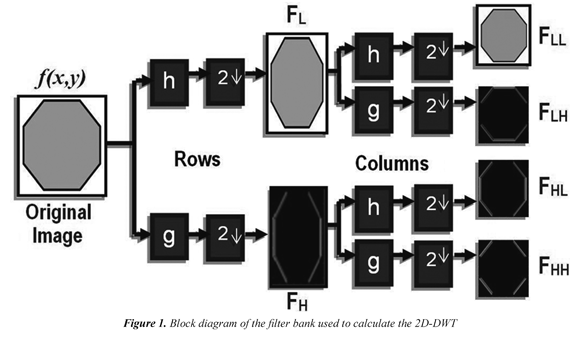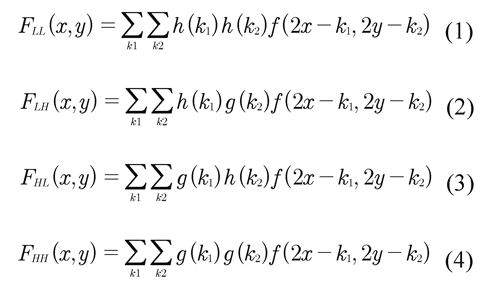Where (x, y) represents the coordinates of the images, h and g are the high pass and low pass filters respectively, f is the input image and k represents the size of the filters.. The process can be iterated to higher levels, assuming the average image FLL as input for the next level. Figure 2 shows the transforming for two levels.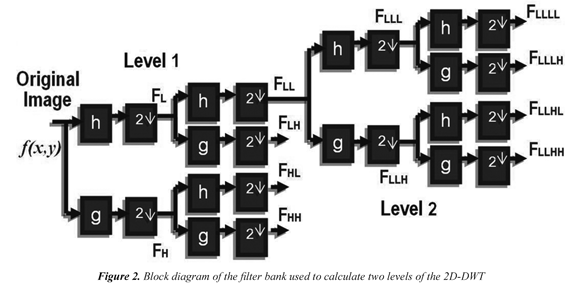The architecture presented in this article is adapted to a compression system, however can be used in any application requiring sub-band frequency analysis, according to Ríos (2011), the biorthogonal bases like Bior5.3 and Bior9.3 have coefficients which can be converted to integers using a simple normalization, therefore is not required in the implementation to use modules of floating point arithmetic, in this same work, some results showing that it is possible to use 16-bit integer coefficients of accuracy without significantly affecting the reconstruction quality of image, for that reason the proposed architecture was implemented using integer arithmetic. For that reason the proposed architecture was implemented using arithmetic of integer number. For applications requiring representation in floating point, it is possible maintain the overall structure of the architecture, but it will be necessary to modify the calculation blocks for working floating point arithmetic.

2.1 2D-DWT architectures hardware - state of the art

Chen et al, propose a parallel processing architecture that calculates the 2D -DWT using an adaptation of Recursive Pyramid Algorithm (RPA) Vishwanath (1994). The general idea of the RPA consists in rethinking the order in which the transform coefficients are calculated. So, we are looking for start the calculation of the next level without completing the calculation of the coefficients of the previous level, Chen presents an adaptation to transformations in two dimensions and instead to organize the transformation making the calculus pixel-to-pixel, a planning of rows is done. Therefore the coefficients of an entire row must be calculated in parallel, the effort required to keep track of the last coefficients calculated increases the complexity of the controller becoming its main disadvantage. Vishwanath et al (1994) present two architectures, the first one consists essentially of a one-dimension module for conversion that is used repeatedly to calculate the 2D -DWT, the advantage of the architecture is its simplicity, but it requires too many memory cells which makes it inconvenient for implementation on a chip, another drawback is the latency required to generate the first output data; the proposed second architecture by Vishwanath et al (1995), consists of a systolic filter that handles the filtering in the horizontal direction, a parallel filter to handle the vertical direction and a storage unit. Two rows are processed in the systolic filter in the order of RPA schema while a parallel filter calculates 4 rows which constitutes four outputs of the first level being one of them carried to the systolic filter for further processing, this approach allow improve the performance with respect to the first architecture but the area required for its implementation increases. Colom et al (2001) present an architecture that works with non -separable 2D filters based on a parallel structure called even-odd, the architecture is recurrent.

There is a filter unit which is used for calculating the first level and presents processing continuous, another filter unit is responsible for the calculation of the other levels and begins when the first unit has generated the first four rows and its calculus continue each time that the first generates two new lines; the intermediate time periods are utilized for implementing the recurrence. Two storage units which act as a link between levels are used, the architecture uses distributed control units in order to provide scalability, there is a control inside of the filter unit and a control for synchronizing the operation between two consecutive levels; by increasing the number of levels the number of control units must be replicated. Sheu et al (2000), propose an architecture which involves two horizontal filters modules for calculating the coefficients along rows and two vertical modules for calculating along the columns, each module consists of a high band pass filter and a low band pass filter. A horizontal filter processes the lines and stores the result in the first storage unit, then the result is processed by the vertical filter, the output of the bottom filter is loaded into the horizontal filter and the process is iterated. The filter modules are based on a methodology of reuse of input data with a parallel - pipelined structure. Chakrabarti et al (1999), show two architectures, the first involves two memory units and four parallel filters units composed of a high pass filter and a low pass filter, the first two filters calculate along rows, its output is stored in the first memory unit where data are read by columns for the following two filters and the coefficients are calculated along the columns, similarly the outputs of these filters are stored in the second memory unit by columns and read in rows by the second filter, in the work two scheduling algorithms of the data stream that can be used on this architecture are presented, due to the filter units are recursively used to calculate two sub images, a delay of N cycles is generated which may be unacceptable for some applications. The proposed second architecture by Andra et al (2002 ), is a modified version of the above which seeks to reduce the delay generated by the recursion, this proposal increases two units of filters to produce at the same time the output of all sub images of the same level achieving reduce the size of the storage units and delay.

This article presents an alternative architecture with a simple routing and a control unit of moderate complexity which decreases the time required to compute the discrete wavelet transform in two dimensions.

2.2 2D- DWT approach architecture

Figure 3 shows the block diagram of the proposed architecture for executing the 2D - DWT in hardware, the architecture consists of three storage units (UM1, UM2, UM3), a control unit and three parallel filters units (UF1, UF2 , UF3) composed of a high-pass filter and a low pass filter.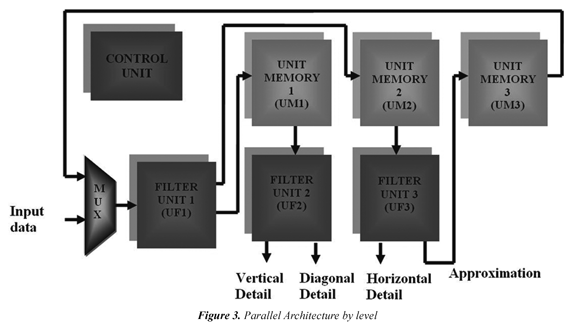The control unit is responsible for scheduling the data flow as follows: the UF1 processes the input image along the rows and generates the intermediate images FH and FL, these sub images are stored in UM1 and UM2 for be carried out to UF2 and UF3 where images are processed by columns, in UF2 the coefficients of the FHL, FHH sub images are generated while in UF3 both FLL and FLH are provided. The output of the low component of UF3 is stored in UM3 to be loaded later in UF1 where the process is iterated to calculate the next level.

The design was implemented on the development board DE2 (2010), this card is composed by an FPGA Cyclone II EP2C35F672C6 (2010) and several storage units (SDRAM, SRAM and FLASH). It is possible to create on the FPGA an instance of the Nios II module for applications that require a processor, the card also involves standard interfaces such as RS- 232 and PS/2, standard connectors for microphone, input and output audio (24 bits), video input (TV Decoder), VGA (10bits DAC), offers USB 2.0 connectivity , Ethernet 10/100 , an infrared port ( IrDA), connectivity to other cards required by the user by means of two expansion modules. For the mentioned reason the card was considered an ideal platform for prototyping regarding multimedia and networks applications. In this work the picture was taken from a file stored in the SDRAM. Figure 4, shows the block diagram of the system implemented on the DE2.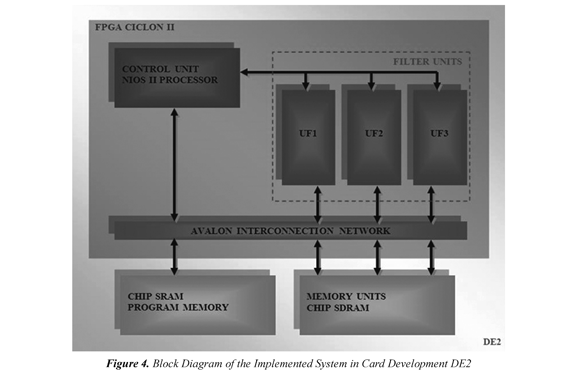2.3 Unit memory

As mentioned in the previous section, the memory units store the data obtained from the processing, the UM1 and UM2 units store the results of the transformation along the rows (FL, FH). NxN/2 memory cells are required for storing the result of processing an image of size NxN. The UM3 unit stores the low frequency component results of the transformation by columns; for that N/2xN/2 cells are required. Therefore the requirements of capacity of memory of the proposed architecture are determined by:The Cyclone II EP2C35F672C6 FPGA has an internal memory structure organized in 3 columns containing a total of 105 blocks that provide a storage capacity of 483840 bits and a maximum operating speed of 250MHz, in consequence it will be able to process images with N lower than 220 pixels, although it is possible to expand the internal storage using blocks of logical arrangements to store data, it is not recommended since only increase 2047 bytes using all the resources of the FPGA. To process larger images, storage capacity of the system was increased by using one of the DE2´s block memory. The SDRAM IS42S16400 which stores data of 8 Mbytes was used; respect to interface connection, Altera has developed a tool called SoPC builder (System expanded on a Programmable Chip) (2010 ), which allows reuse IP blocks and uses the AVALON interconnect bus which requires less logic elements in the connection and improves performance in the transmission rate.

2.4 Filters unit

The filter unit is based on a reuse methodology of input data mixed with a parallel-pipelined structure similar to that proposed by Sheu et al (2000), but the calculation of the coefficients is performed using the methodology even-odd reported by Colom et al (2001). This strategy allows the calculation of one coefficient by cycle after 2 cycles of latency, the overall scheme of the filter unit is shown in Figure 5. Each unit has two filters, and each filter is composed of: a shift register that stores the filter coefficients and is configured so that each cycle makes two shifts; three units of Multiplier-Adder-Accumulator (MSA1, MSA2, MSA3) composed of two multipliers (one for pair data and another for the odd data); an adder and an accumulator register; a multiplexer that selects which of the data is ready to be sent to the next stage.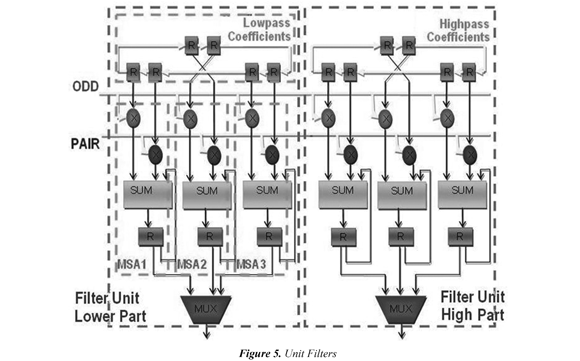To illustrate the operation of this unit, we must consider two filters h (Low-pass) and g (High-pass) with six coefficients defined as:Let f be a signal with N data, whose discrete values are defined so: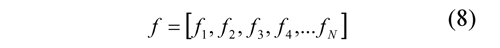By transforming the signal f using the filters g and h, two signals are obtained, one of approximation (A) and another with the details (D), whose coefficients can be expressed as follows: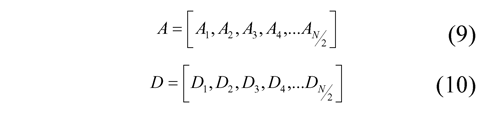Considering that the one-dimensional transformation is defined by the following equations: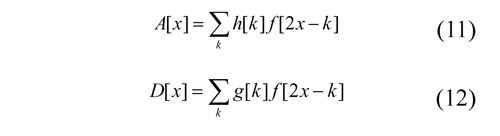We can use Eq. (11) to express each coefficient as follows: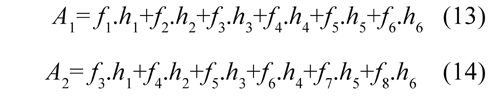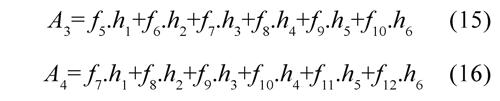To reach the limits of the signal where: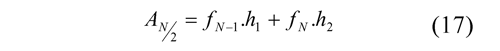Reuse methodology consists in organizing the flow of the input data so that it can be calculated in parallel several output data, this process is illustrated in Figure 6.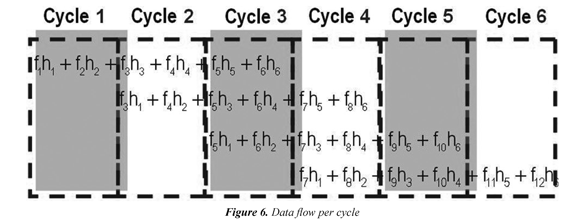In each cycle the register of coefficients shifts two spaces. So in the first cycle, to MSA1 arrives the coefficients h1, h2; to MSA2 arrives h3, h4 and to MSA3 arrives h5, h6. In the second cycle to MSA1 arrives the coefficients h3, h4; to MSA2 arrives the coefficients h5, h6 and to MSA3 arrives and h1, h2. In the third cycle, to MSA1 arrives the coefficients h5, h6; to MSA2 arrives h1, h2 and to MSA3 arrives h3, h4. The process repeats until the end of the transformation. In the first cycle enters f1, f2, with these data the MSA1 begins the calculation of A1 accumulating f1.h1 + f2.h2. In second cycle enters f3, f4, so the MSA1 accumulates f3.h3 + f4.h4 while in parallel the MSA2 uses the same input data to calculate A2 accumulating f3.h1 + f4.h2. In the third cycle enters f5, f6, the MSA1 unit calculates f5.h5 + f6.h6 thereby completing the calculation of A1. In parallel, MSA2 calculates f5.h3+ f6.h4 and MSA3 begins the calculation of A3 accumulating f5.h1+ f6.h2.

Henceforth, one output data will be provided in each cycle while the multiplexer turns on in order to lead this result to the output. In the fourth cycle enters f7,f8, the MSA1 begins the calculation of A4 accumulating f7.h1+f8.h2, the MSA2 calculates f7.h5+f8.h6 completing thus the calculation of A2, the MSA3 accumulates f7.h3+f8.h4. In the fifth cycle enters f9,f, the MSA1 accumulates f9.h+f, the 10310.h4 MSA2 begins the calculation of A5 and the MSA3 completes the calculation of A3 accumulating f9.h+f; hereinafter the process is iterated until 510.h6 the end of the input signal.

Following the methodology described in the previous paragraph, the components of high pass of the output signal must be calculated in a parallel architecture, the only difference lies in the stored data in the shift register which must correspond to the coefficients of the high pass filter. Note that each input data is used in the partial calculation of several output coefficients.

When processing the pairs in parallel with the odd two output data (one high pass and one low-pass) are available at each clock cycle after the third cycle. Thus a one-dimensional signal transforms of size N is performed in N/2 + 2 clock cycles, by expanding the processing for two-dimensional signal of size N x N requires (N/2 + 2)N cycles to process all rows and (N/2 + 2 )N/2 cycles to process all columns, thus the total of required cycles for the proposed architecture for computing the coefficients of the first level of transformation is determined by:The filter unit was modeled using VHDL and Altera megafunciones, structural design has 5 modules: Registry coefficients, multiplier, adder, accumulator register and multiplexer. For the implementation of the multipliers of lpm_mul Altera megafunción allowing embedded multipliers used by optimizing the use of the FPGA, for the megafunción Altera Multiplexer lpm_mux to synthesize as described in VHDL design 17 required logical elements are used further, the adder module was implemented with the megafunción parallel_add as described in VHDL design required a longer stabilization 0.577ns, the remaining elements and the connecting lines were modeled in VHDL.

This unit was synthesized in a Cyclone II FPGA of Altera EP2C35F672C6 using the Quartus II version 6.0 design web edition, Table I shows the resources used in the implementation of the filter unit.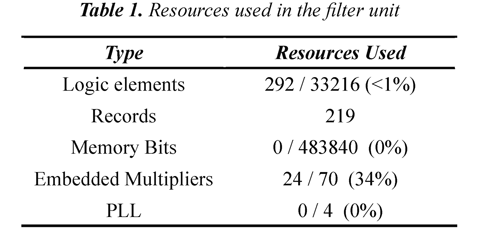Table I notes that in the implementation of the filter unit 34% of embedded multipliers, 1% of the logic elements and 0 % of bits of memory are used, this is evidence that space is available within FPGA to increase the degree of parallelism in the design and simultaneously use multiple filter units , which would provide the coefficients for higher levels in less time occupying all the available multiplier, in applications where FPGA is used only for implementation phase of this transformation is a viable option, but in applications where you need to implement additional functions on the FPGA using the recursive filter units for the calculation of the following levels of transformation is necessary.

Figure 7 shows part of the results of the simulation of the filter unit, in the first clock cycle of the charging records the values of coefficients of the filters is performed using the charge control signal (Load) records storage and cleaning using the signal (Clear) in the second cycle partial operations is cleaned, from the third cycle output two coefficients are obtained in each cycle for which multiplexers are switched through its signal selection (Sel), three cycles are necessary to generate signals cleaning climbing to clear accumulators records, pairs data are represented by the signal called FPAR and odd by Fimpar, the coefficients of high frequency transformation signal output a and D low frequency, for the validation of the data obtained the system model in Matlab and the results were compared, the data obtained from the processing and processed in Matlab of the simulation in Quartus totally agree (Error 0 % ), which is consistent with expectations since at this stage the data have not yet been quantified.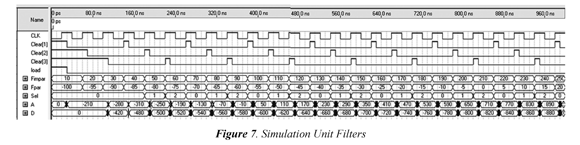2.5 Control unit

For the architecture control-unit, a design which is supported in the NIOS II  system is used. Nios II is defined in a hardware description language which can be implemented in Altera FPGAs using Quartus II synthesis tool in conjunction with the processor SoPC Builder, there are three versions of the processor: the economic Nios/e, the standard Nios/s and rapid Nios/f. The economic version has a smaller core this version does not handle cache memory or specialized hardware to develop arithmetic operations; for the control architecture, the economic version was chosen because the operations required to control the architecture does not include complex operations.

Control instructions are developed in C + + using the integrated development environment NIOS II IDE that enables compile, debug and download fonts in C / C + + on the developed system . To store the program to be executed must allocate a memory space, the DE2 board has a chip that stores 512Kbytes SRAM and is included in the system as program memory (see Figure 4); as well as the SDRAM, the SRAM is connected to the AVALON bus, using the SoPC Builder. The flow chart of the main program is shown in Figure 8 and the flowchart of the function for calculating the transform of a dimension is shown in Figure 9.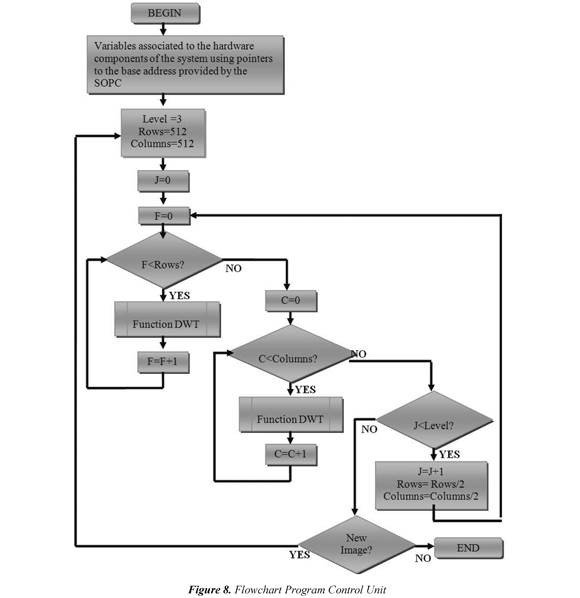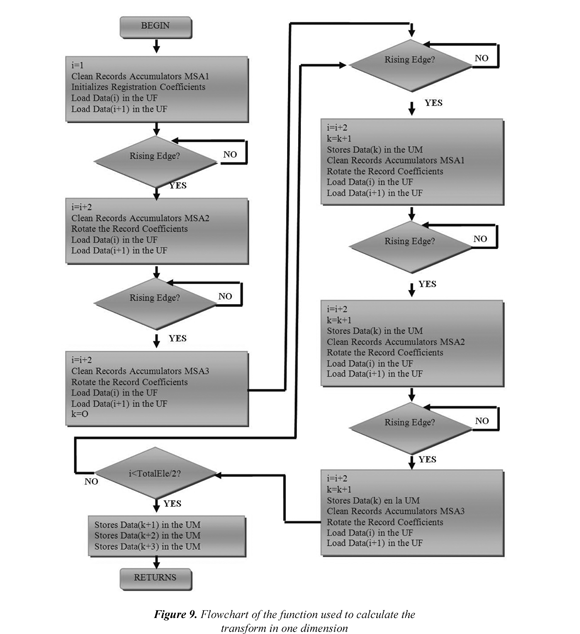Calculation blocks access memory-units through the processor, it generates the instructions to connect to an interface block which permits access of the SDRAM through a bus Avalon, the processor or control unit plans all data flow through the architecture generating the necessary signals for operation ( load signals to the records, select signals for the multiplexers, read and write signals on the SDRAM, etc.)

3. Results and discussion

In architectures implemented on FPGAs, it should be taken into account that they contain a limited number of logic cells. Therefore, it is necessary that the design is oriented towards the optimization of this resource. Whereas a large number of logic cells is required for implementing storage elements, it is convenient to use a lower requirement architectures memory. Similarly routing complexity also leads to a high consumption of logic cells because more elements are required to interconnect the system being desirable a less complex routing network; another major factor is the time required for the calculation of the transformation, it should be minimized to process large amounts of images at satisfactory rates, and likewise it is desirable to reduce the complexity of the control system so that the architecture is easily scalable and programmable. Achieving these objectives is particularly difficult in an FPGA as area and speed are inversely proportional and satisfying a requirement means significantly affecting the other, for example, the speed can be increased with a high degree of parallelism which implies an increase in the required area. Table II relates these features to the surveyed and proposed architectures from Table II can be observed that the proposed architecture in this paper presents a decrease in computation time compared to the surveyed architectures, in the most notable case is improved approximately 3N2 cycles and the least significant event is approximately 0.25N2 cycles, the improvement does not complicate implementation since a simple routing is maintained and control unit with moderate complexity is preserved . If in fact in our proposal required more store cells, this mishap can be overcome by using an external memory for data storage.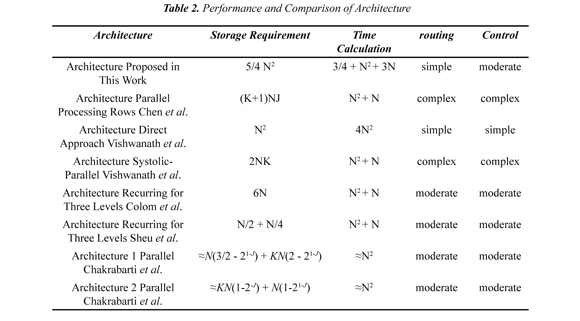4. Conclusion

The hardware architecture presented to develop the discrete wavelet transform in two dimensions provides an efficient performance and speed of calculation area, the architecture uses 3/4N2 +2 N cycles for transforming an image of size NxN achieving improvement over architectures developed on previous works, also it maintains control and a routing of moderate complexity. This architecture is adapted to an image processing system, however it can be used in any application of signal requiring subband frequency analysis.

5. References

Altera Corporation (2010). Cyclone II Device Handbook . Recovered 2014/06/15 http://www.altera.com        [ Links ]

Altera Corporation. (2010). User Guide SoPC Builder . Recovered 2014/06/15 http://www.altera.com/literature/lit-nio2.jsp#related_documentation        [ Links ]

Altera Corporation. (2011). Nios II Processor Reference Handbook . Recovered 2014/06/15 http://www.altera.com/literature/lit-nio2.jsp#related_documentation        [ Links ]

Andra, K., Chakrabarti, C., & Acharya, T. (2002) A VLSI architecture for lifting-based forward and inverse wavelet transform. IEEE Transactions on Signal Processing , 50 (4), 966-977.         [ Links ]

Chakrabarti, C., & Mumford, C. (1999). Efficient Realizations of Encoders and Decoder Based on the 2-D Discrete Wavelet Transform. IEEE Transactions on Very Large Scale Integration (VLSI) Systems , 7 (3), 289-298.         [ Links ]

Chen, C., Yang, Z., Wang, T., & Chen, L. (2000). A programmable VLSI architecture for 2-D discrete wavelet transform. In IEEE International Symposium ON Circuits and Systems (pp. 619-622).         [ Links ]

DE2 User Manual, Altera Corporation. (2010). Recovered 2014/06/15 ftp://ftp.altera.com/up/pub/        [ Links ]

Colom, R. J., Gadea, R., Sebastiá, A., Martinez, M., Ballester, F., & Herrero, V. (2001). Implementación de la Transformada Wavelet Discreta 2D con filtros no separables. In I Conference on Reconfigurable Computing and Applications . Alicante, Spain.         [ Links ]

Colom, R. J., Gadea, R., Sebastiá, A., Martinez, M., Herrero, V., & Arnau, V. (2001). Transformada Discreta Wavelet 2-D para Procesamiento de Vídeo en Tiempo Real. In XII Parallelism Workshop . Valencia, Spain.         [ Links ]

Grgic, S., & Grgic, M. (2001). Performance Analysis of Image Compression Using Wavelets. IEEE Transactions on Industrial Electronics, 48 (3), 682-695.         [ Links ]

Hilton, M.L., Jawerth, B.D., & Sengupta, A. (1994). Compressing still and moving images with wavelets. Multimedia Systems , 2 (3), 218-227.         [ Links ]

Ríos, X. (2011). Diseño e Implementación de un Sistema de Compresión de Imágenes Usando Dispositivos Reprogramables . Master Thesis, Faculty of Engineering, Universidad del Valle, Cali, Colombia.         [ Links ]

Ríos, X., & Bernal, A. (2011). Implementación de un Sistema de Compresión en el Dominio Wavelet sobre una FPGA Usando el Procesador Nios II. In II International Congress on Instrumentation Control and Telecommunications . Tunja, Colombia.         [ Links ]

Sheu, M., Shieh, M., & Liu, S. (1998). A VLSI Architecture Design With Lower Hardware Cost and Less Memory for separable 2-D Discrete Wavelet Transform. In IEEE. Proceedings of the IEEE International Symposium on Circuits and Systems (ISCAS) (vol.5) (pp. 457-460). Monterrey (CA): IEEE.         [ Links ]

Vishwanath, M. (1994). The Recursive Pyramid Algorithm for the discrete wavelet transform. IEEE Transactions on Signal Processing , 42(3), 673-676.         [ Links ]

Vishwanath, M., Owens, R. M., & Irwin, M. J. (1995). VLSI architectures for the discrete wavelet transform. IEEE Transactions on Circuits and Systems - II , 42 (3), 305-316.         [ Links ]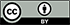Revista Ingeniería y Competitividad por Universidad del Valle se encuentra bajo una licencia Creative Commons Reconocimiento - Debe reconocer adecuadamente la autoría, proporcionar un enlace a la licencia e indicar si se han realizado cambios. Puede hacerlo de cualquier manera razonable, pero no de una manera que sugiera que tiene el apoyo del licenciador o lo recibe por el uso que hace.